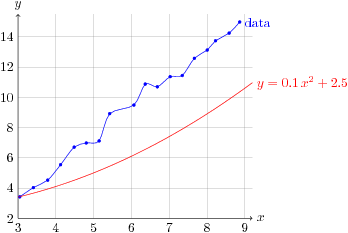## Creating plots in LaTeX with Gnuplot and Tikz

Plotting data in LaTeX is a useful thing to do. Xavier Delaunay shows how to do it on his homepage:

http://d.xav.free.fr/tikz/index.html

The plot looks like this:## Really easy 3D graphics in LaTeX

Note: The word "easy" is relative to the other solutions I found for doing 3D graphics in LaTeX.

The following template is adapted from the annotated 3d box example.

% Template for drawing with tikz 3D package \documentclass[]{article} \usepackage{tikz} \usetikzlibrary{3d}   \begin{document}   % Draw a 3D coordinate system   \begin{center} \begin{tikzpicture}[x = {(1cm,0cm)}, y = {(0.4cm,0.6cm)}, z = {(0cm,1cm)}, scale = 1, color = {lightgray}]   \begin{scope}[canvas is xy plane at z=0] % x-axis \draw[blue,->] (0,0) -- (5,0) node (x-axis) [right] {$x$}; % y-axis \draw[red,->] (0,0) -- (0,5) node (y-axis) [above] {$y$}; \end{scope}   \begin{scope}[canvas is yz plane at x=0] % z-axis \draw[green,->] (0,0) -- (0,5) node (z-axis) [above] {$z$}; \end{scope}   \end{tikzpicture} \end{center} \end{document}

## tikZ and LaTeX

PGF/TikZ is a tandem of languages for producing vector graphics from a geometric/algebraic description. You can use this language inside tex files, to programmatically draw nice 2D and 3D graphics. Another package which some people prefer for 3D is asymptote.

\usepackage{multicol} \usepackage{blindtext}
\begin{multicols}{2}[\textbf{Example for a two column text}] \blindtext \end{multicols}